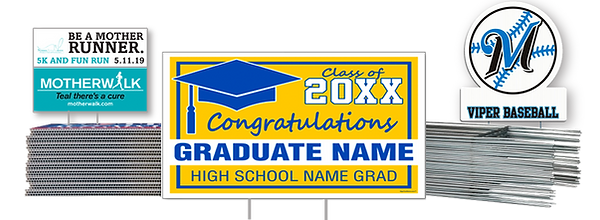top of page1/4 COROPLAST, OUTDOOR, INDOOR YARD SIGNS WITH STEP STAKE.  18 X 24"

PRINTED 1 SIDE

5 = \$15.00 EA

10 = \$13.00

30 = \$11.50

50 = \$ 9.75

100 = \$8.75

150 = \$7.75

200 = \$6.75

PRINTED 2 SIDES

5 = \$25.00 EA

10 = \$22.00

30 = \$16.50

50 = \$13.75

100 = \$11.75

150 = \$10.75

200 = \$  8.75

bottom of page